# Contractible space

This article defines a homotopy-invariant property of topological spaces, i.e. a property of homotopy classes of topological spaces

View other homotopy-invariant properties of topological spaces OR view all properties of topological spaces

## Definition

### Equivalent definitions in tabular format

A nonempty topological space is said to be contractible if it satisfies the following equivalent conditions. The empty space is generally excluded from consideration when considering the question of contractibility.

No. Shorthand A topological space is termed contractible if ... A topological space$X$ is termed contractible if ...
1 homotopy-equivalent to a point there is a homotopy equivalence of topological spaces between the topological space and a one-point space. There exist continuous maps$f: X \to \{ * \}$ and$g: \{ * \} \to X$ such that$f \circ g$ is homotopic to the identity map on$\{ * \}$ and$g \circ f$ is homotopic to the identity map on$X$. Here,$\{ * \}$ is a one-point space. Also, note that the condition on$f \circ g$ is tautological, and the map$f$ is already uniquely determined, so all the action occurs in the definition of$g$ and the homotopy between$g \circ f$ and the identity map on$X$.
2 homotopy-equivalent to a point (arbitrary map) any pair of maps between the space and a one-point space define a homotopy equivalence of topological spaces. For any continuous map$f: X \to \{ * \}$ and$g: \{ * \} \to X$,$f \circ g$ is homotopic to the identity map on$\{ * \}$ and$g \circ f$ is homotopic to the identity map on$X$. Here,$\{ * \}$ is a one-point space. Also, note that the condition on$f \circ g$ is tautological, and the map$f$ is already uniquely determined, so all the action occurs in the definition of$g$ and the homotopy between$g \circ f$ and the identity map on$X$.
3 admits a contracting homotopy there is a point in the space to which there is a contracting homotopy. there exists a point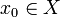$x_0 \in X$ and a contracting homotopy that contracts$X$ to$x_0$. Explicitly, there exists a continuous map$F: X \times [0,1] \to X$ such that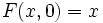$F(x,0) = x$ for all$x$, and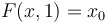$F(x,1) = x_0$ for all$x$. Note that we do not assume or require that$F(x_0,a) = x_0$ for all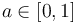$a \in [0,1]$.
4 admits a contracting homotopy (arbitrary point) the space admits a contracting homotopy to any point in it. for any point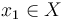$x_1 \in X$, there is a contracting homotopy that contracts$X$ to$x_1$.
5 unique homotopy class of maps to it for any other topological space, there is a unique homotopy class of maps from the other space to it. for any topological space$Y$, and any two continuous maps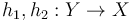$h_1, h_2: Y \to X$,$h_1$ and$h_2$ are homotopic. In particular, any map from a topological space to it is nullhomotopic.
6 retract of cone space it is a retract of its cone space. the inclusion$\iota: X \to CX$ in the cone space$CX$ (as the base) has a one-sided inverse,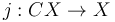$j: CX \to X$, i.e.,$j$ is a continuous map such that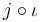$j \circ \iota$ is the identity map on$X$.

## Metaproperties

Metaproperty name Satisfied? Proof Statement with symbols
product-closed property of topological spaces Yes contractibility is product-closed If$X_i, i \in I$ form a (finite or infinite) collection of contractible spaces, then the product of the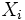$X_i$s, equipped with the product topology, is also contractible.
In particular, if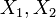$X_1, X_2$ are contractible, then$X_1 \times X_2$ is also contractible.
retract-hereditary property of topological spaces Yes contractibility is retract-hereditary If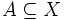$A \subseteq X$ and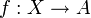$f:X \to A$ is a continuous retraction, and$X$ is contractible, then$A$ is contractible.
suspension-closed property of topological spaces Yes contractibility is suspension-closed The suspension of a contractible space is contractible.
closure-preserved property of topological spaces No contractibility is not closure-preserved It is possible to have a topological space$X$ and a subset$A$ of$X$ such that$A$ is contractible in the subspace topology, but the closure$\overline{A}$ is not.
connected union-closed property of topological spaces No contractibility is not connected union-closed It is possible to have a topological space$X$ expressible as a union of subsets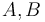$A,B$, both contractible in their subspace topology, with$A \cap B$ nonempty, but$X$ itself not contractible.

## Examples

### Intuition behind examples

Contractibility is, fundamentally, a global property of topological spaces. It is not possible to examine a small part of the space and conclude that it is contractible, nor does examining a small part of the space allow us to rule out the possibiilty that it is contractible. For the intuition behind the former, note that we can attach non-contractible pieces (like circles) far off from the part of the space we are looking at. For the intuition behind the latter claim, note that we can embed any topological space as a closed subspace of a contractible space, namely, its cone space.

For this reason, when looking for examples or counterexamples, we need to focus on the global structure.

### Examples from topological construction

One thing to keep in mind is that since the definition of contractibility invokes the closed unit interval, it is likely that any effort to construct contractible spaces will invariably involve dealing with the real numbers. The most topologically general way of constructing a contractible space is as the cone space of an arbitrary topological space. One way of thinking of this cone space is as a literal cone that fills in between the space and a point. Up until the very tip of the cone, the cross-sections look homeomorphic to the topological space.

### Examples from geometry

A topologically star-like space is a classic example of a contractible space. A topological space is termed topologically star-like if it is homeomorphic to a star-like subset of Euclidean space. A star-like subset of Euclidean space is a subset for which there exists a point in it such that for every other point in it, the line segment joining the points is completely inside the space.

A topologically star-like space is contractible, and can in fact be contracted to any point relative to which it is a star through a straight-line homotopy, i.e., moving each point toward the center in a straight line. The contracting homotopy fixes the center, and therefore, the space is in fact a SDR-contractible space.

Note that, if also compact, a topologically star-like space is homeomorphic to the cone space of its boundary. Otherwise, the space is still almost a cone space: it is a subspace of the cone space that contains the full complement of the base and an arbitrary subset of the base. Nonetheless, it is important to note that the condition of being star-like also carries various geometric implications (in particular, from being a sub-Euclidean space) that are not satisfied for arbitrary cone spaces.

A topologically convex space is a (non-empty) space that is homeomorphic to a convex subset of Euclidean space. Any topologically convex space is topologically star-like, and any point can be taken as the center. An example of a topologically star-like space that is not topologically convex is a pair of intersecting lines.

It is possible to construct spaces that are not topologically star-like, but still contractible. For instance, any geometric realization of a tree is contractible, but if the tree has more than one point with degree greater than two, it is not topologically star-like. As a related example, a set of parallel lines combined with one line that intersects all of them form a contractible space that is not topologically star-like.

## Relation with other properties

### Incomparable properties

Contractibility is incomparable with most of the interesting separation and compactness properties.

Broad argument for why contractibility cannot imply any meaningful separation or compactness property: The cone space over any topological space is contractible. In particular, since any topological space arises as a closed subspace of its cone space (namely, the "base" of the space), every topological space arises as a closed subspace of a contractible space. Therefore, contractible cannot imply any nontrivial property that is subspace-hereditary or even weakly hereditary (inherited by closed subsets).

Broad argument for why meaningful separation or compactness properties cannot imply contractibility: Most meaningful separation and compactness properties are satisfied by all compact manifolds. However, compact manifolds of dimension greater than one are not contractible. The simplest counterexample is generally the circle.

Some incomparable properties:

The property of being a contractible space is also incomparable with the property of being a locally contractible space. A contractible space need not be locally contractible. In fact, it need not even be locally connected! An example of a contractible space that is not locally contractible is the comb space. An example of a space that is locally contractible but not contractible is the circle (or, more generally, any compact manifold).

### Stronger properties

Property Meaning Proof of implication Proof of strictness (reverse implication failure) Intermediate notions
cone space over some topological space cone space implies contractible
topologically convex space homeomorphic to a convex subset of Euclidean space via star-like Space in which every retraction is a deformation retraction, Topologically star-like space|FULL LIST, MORE INFO
suddenly contractible space has a contracting homotopy that is also a sudden homotopy |FULL LIST, MORE INFO
SDR-contractible space has a contracting homotopy that is also a deformation retraction |FULL LIST, MORE INFO

### Weaker properties

Property Meaning Proof of implication Proof of strictness (reverse implication failure) Intermediate notions
weakly contractible space path-connected space, all homotopy groups vanish contractible implies weakly contractible weakly contractible not implies contractible |FULL LIST, MORE INFO
multiply connected space path-connected space, first$k$ homotopy groups vanish for$k \ge 2$ the$n$-sphere$S^n$ is$(n-1)$-connected but not$n$-connected. |FULL LIST, MORE INFO
simply connected space path-connected space, fundamental group is trivial Weakly contractible space|FULL LIST, MORE INFO
path-connected space there is a path between any two points Weakly contractible space|FULL LIST, MORE INFO
connected space cannot be partitioned into disjoint nonempty subsets |FULL LIST, MORE INFO
acyclic space homology groups over$\mathbb{Z}$ all zero except zeroth homology group which is$\mathbb{Z}$ Weakly contractible space|FULL LIST, MORE INFO
rationally acyclic space homology groups over$\mathbb{Q}$ all zero except zeroth homology group which is$\mathbb{Q}$ Acyclic space, Weakly contractible space|FULL LIST, MORE INFO
space with Euler characteristic one Euler characteristic of the space is one. (via acyclic) Euler characteristic one not implies acyclic Acyclic space|FULL LIST, MORE INFO